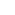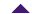## HelpCalculation Variables

Principal
Enter the initial value of the investment here.

Loan Value
Enter the initial value of the loan here.

Interest Rate
In this field, insert the interest rate to be used in the calculation for period defined.

Interest Period
Enter the start and end date of the investment period in these fields. Note that each date is calculated from 00:00:00 of the start date until 23:59:59 of the end date.
Examples:

• From 1998, Jul 1 until 1998, Jul 1 = 1 day
• From 1998, Jul 1 until 1998, July 31 = 1 month
• From 1998, Oct 28 until 1999, Oct 27 = 1 year

Loan Period
Enter the start and end date for the borrowing period in these fields. Note that each date is calculated from 00:00:00 of the start date until 23:59:59 of the end date.
Examples:

• From 1998, Jul 1 until 1998, Jul 1 = 1 day
• From 1998, Jul 1 until 1998, Jul 31 = 1 month
• From 1998, Oct 28 until 1999, Oct 27 = 1 year

Periodic Contributions
Value: Enter the amount of each periodic (i.e. monthly, daily) contribution or payment.
Frequency: How often each contribution is made.

Compound Frequency
Use this field to define how often interest will be earned on your investment or loan.Calculations

Calculation: Investments/Savings
These calculations produce the final value of the principal plus the interest generated on the principal. It is normally used for calculating the value of bonds or stocks over a given period. It can also be used for planning retirement or educational savings.

Calculation: Loans
Given the value of the loan, the interest rate and a period of time and, possibly, the value of Periodic Contributions (e.g., monthly), the total value of the loan plus the interest is calclated. It is used for calculating student loans, mortgages and car loans/leases.

Calendar Dates
This calculator allows the user to select interest periods by exact calendar dates.

Time Periods
The user can select the interest periods by specifying time durations such as a number of years, months, days or hours.Currency Type

0 decimals
For example, Peso, Yen.

2 decimals
For example, Dollar, Pound.

3 decimals
For example, Euro.

Fixed
The interest rate remains the same throughout the entire savings or borrowing period.

Floating
The interest rate varies throughout the evaluation period. You must be able to provide the exact period of each interest rate in order to generate correct results. With DeLiberation� Financial Calculator, you can use an unlimited number of interest rates throughout the evaluation period.Interest Type

Simple
Interest is only generated from the principal value of the loan. Sometimes it is also referred to as "regular" or "non-compounded" interest.

Compound
Interest is generated from both the principal and the interest compounded with a given frequency (e.g. daily). In the case of a loan, interest is generated only on what is still owed, not the entire loan value. Interest for the majority of investment certificates and loans are calculated in this manner.Interest Style

Nominal
A nominal rate is the actual raw number that gets used in each calculation. For example, if we have a 10% nominal annual rate, and we wish to compound monthly ... then the actual monthly rate that will be used is simply (.10/12). Although due to the multiple compouding, by the end of each term the interest accumulated will actually be slightly more than 10% worth.

Effective
An effective rate represents the true and exact amount of interest that will be generated at the end of each term. For example, if we have an effective annual rate of 10%, and we wish to compound monthly ... then the actual monthly rate that will be used is (.096/12) - not simply (.10/12) because with an effective rate, once all of the compounding is completed for the term, the interest will directly reflect a single compound of 10% (or any choosen rate).Periodic Contributions (or Payments)

This calculator has the capability to take into account payments or contributions made on a periodic basis. Note that all payments are assumed to be made at the end of each payment period, and do not include an initial payment at the beginning of the investment/loan.Calculation Formulas Used

Investments/Savings -- Simple Interest -- Fixed Interest Rate

Final Value = (1 + Principal) * Interest Rate * (Interest Period/1 year)

Investments/Savings -- Simple Interest -- Fixed Interest Rate -- Periodic Contributions

Number of Periods = Interest Period/Frequency of Periodic Contributions
For every Period:
Interest += (Principal) * Interest Rate * (Length of Period/1 year)
Final Value = Interest + Principal

Investments/Savings -- Compound Interest -- Fixed Interest Rate

Number of Periods = Interest Period/Compound Frequency
For every Period:
Interest += (Principal) * Interest Rate * (Length of Period/1 year)
Principal += Value of Periodic Contributions
Final Value = Interest + Principal

Investments/Savings -- Compound Interest -- Fixed Interest Rate -- Periodic Contributions

Number of Periods = Interest Period/Frequency of Periodic Contributions INT Interest Period/Compound Frequency
For every Period:
Interest += (Principal) * Interest Rate * (Length of Period/1 year)
Final Value = Interest + Principal

Investments/Savings -- Simple Interest -- Floating Interest Rate

For each Interest Rate:
Interest += (Principal * Interest Rate) * (Interest Period/1 year)
Final Value = Interest + Principal

Investments/Savings -- Simple Interest -- Floating Interest Rate -- Periodic Contributions

For each Interest Rate:
Number of Periods = Interest Period/Frequency of Periodic Contributions
For every Period:
Interest += (Principal) * Interest Rate * (Length of Period/1 year)
Principal += Value of Periodic Contributions
Final Value = Interest + Principal

Investments/Savings -- Compound Interest -- Floating Interest Rate

For each Interest Rate:
Number of Periods = Interest Period/Compound Frequency
For every Period:
Interest += (Principal * Interest Rate) * (Length of Period/1 year)
Final Value = Interest + Principal

Investments/Savings -- Compound Interest -- Floating Interest Rate -- Periodic Contributions

For each Interest Rate:
Number of Periods = Interest Period/Frequency of Periodic Contributions INT Interest Period/Compound Frequency
For every Period:
Interest += (Principal * Interest Rate) * (Length of Period/1 year)
Principal += Value of Periodic Contribution
Final Value = Interest + Principal

Loans -- Simple Interest -- Fixed Interest Rate

Final Value = (1 + Loan Value) * Interest Rate * (Interest Period/1 year)

Loans -- Simple Interest -- Fixed Interest Rate -- Periodic Contributions

Number of Periods = Interest Period/Frequency of Periodic Contributions
For every Period:
Interest += (Loan Value) * Interest Rate * (Length of Period/1 year)
Loan Value -= Value of Periodic Contribution
Final Value = Interest + Loan Value

Loans -- Compound Interest -- Fixed Interest Rate

Number of Periods = Interest Period/Compound Frequency
For every Period:
Interest += (Loan Value) * Interest Rate * (Length of Period/1 year)
Loan Value += Value of Periodic Contributions
Final Value = Interest + Loan Value

Loans -- Compound Interest -- Fixed Interest Rate -- Periodic Contributions

Number of Periods = Interest Period/Frequency of Periodic Contributions INT Interest Period/Compound Frequency
For every Period:
Interest += (Loan Value) * Interest Rate * (Length of Period/1 year)
Loan Value -= Value of Periodic Contributions
Final Value = Interest + Loan Value

Loans -- Simple Interest -- Floating Interest Rate

For each Interest Rate:
Interest += (Loan Value * Interest Rate) * (Interest Period/1 year)
Final Value = Interest + Loan Value

Loans -- Simple Interest -- Floating Interest Rate -- Periodic Contributions

For each Interest Rate:
Number of Periods = Interest Period/Frequency of Periodic Contributions
For every Period:
Interest += (Loan Value) * Interest Rate * (Length of Period/1 year)
Loan Value -= Value of Periodic Contributions
Final Value = Interest + Loan Value

Loans -- Compound Interest -- Floating Interest Rate

For each Interest Rate:
Number of Periods = Interest Period/Compound Frequency
For every Period:
Interest += (Loan Value * Interest Rate) * (Length of Period/1 year)
Final Value = Interest + Loan Value

Loans -- Compound Interest -- Floating Interest Rate -- Periodic Contributions

For each Interest Rate:
Number of Periods = Interest Period/Frequency of Periodic Contributions INT Interest Period/Compound Frequency
For every Period:
Interest += (Loan Value * Interest Rate) * (Length of Period/1 year)
Loan Value -= Value of Periodic Contribution
Final Value = Interest + Loan Value